October 22, 2015

If you need to add leading zeros to a value in Excel there are two methods that you can use, depending on whether the result you want is a numeric or text value. To find out how to add leading zeros to a value in Excel read on to discover how both methods are used.

There are many reasons why you might need to add leading zeros to a value in Excel, one that springs to mind is the creation of employee ID’s or membership ids. Sometimes having a standardised format, such as every ID number being a length of 6, is useful for long-term management of large lists.

For example, your ID’s might currently look like this: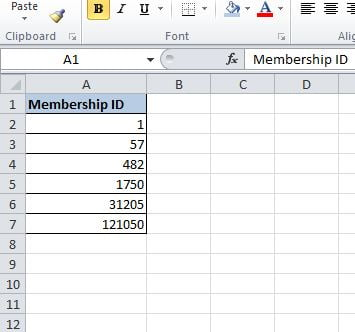But standardised into a 6 digit length would show them like so: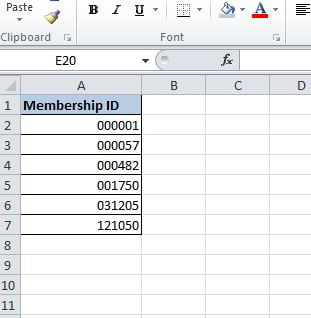There are two ways you can achieve adding leading zeros to a value in Excel. The first is by formatting the original values; this is the quickest way and ensures they remain numeric.

To format the values first highlight the values you want to format, starting in the first cell and holding the left mouse button down drag the cursor to the end of the range so they all become highlighted: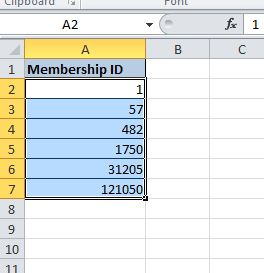Then right click the mouse anywhere within that highlighted range, from the pop-up window select “Format Cells”:The next step is to tell Excel how to format the cells. In the Format Cells window that opens up do the following:

1) Select the Number tab

2) Select the Custom Category

3) Change the Type box to contain six zeros, this is telling Excel to format the length to six and where the length is shorter pad with leading zeros: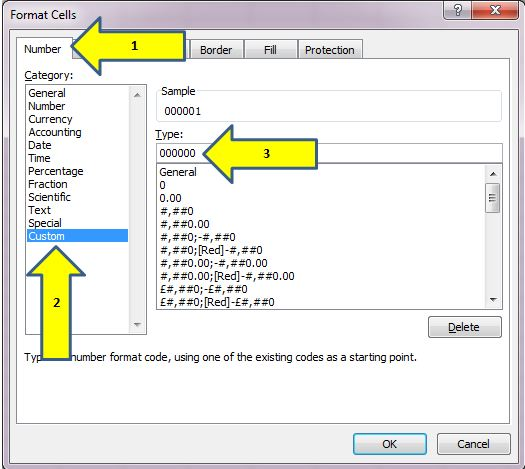When you have completed this click on OK and the values you highlighted will have now changed to the new format: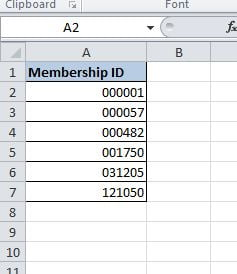The Other Way – Make them text

The second way is creating a new value using a formula. This will result in the membership IDs becoming a text value rather than a numeric so if you need text values then this is the approach to take. This method is more complicated than a straight-forward format but something even a novice Excel user can fully understand with the following explanation.

The first function that will assist with the task is the REPT function.

The REPT function repeats text a specified amount of times and takes the format:

=REPT(text, number_times)

This is where text is the value you want to repeat and number_times is the amount of times you want to repeat the text. So to generate six zeros the formula would look like:

=REPT(0,6)

That alone does not solve the original problem; a twist is required to make it convert the existing membership IDs into six digits in length.

Consider the problem

The problem is that some of the values are one digit in length, some are already six, and there is also a mixture of lengths in-between:Also, the REPT function is just generating six zeros, it is not currently doing anything with the existing membership ID’s: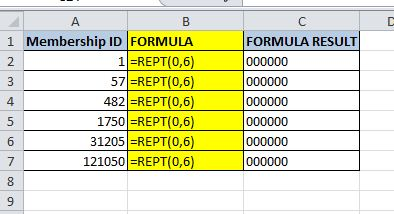The Solution

To resolve the first thing to do is add the leading zeros to the existing membership ID, to do so add the cell reference to the end of the formula using the ampersand (;), like so: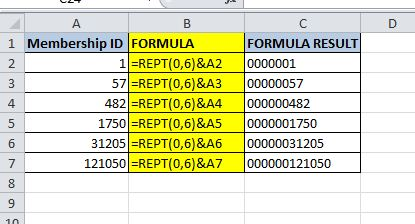Now there are six leading zeros before each membership ID, but the aim is to have them all six digits in length. The key here is the length of the value, this is where Excels LEN function will help set everything to six digits.

The LEN Function returns the number of characters in a string; effectively it tells Excel how long the string is. This information can be used to tell Excel how many zeros it needs to add, depending on the current length of the string. For example, if the membership ID is 482 then we already have a length of three digits so we only want to add three leading zeros, therefore the existing formula just needs the length of the existing value subtracted from a number of zeros:

=REPT(0,6-LEN(A2));A2

Or in Excel: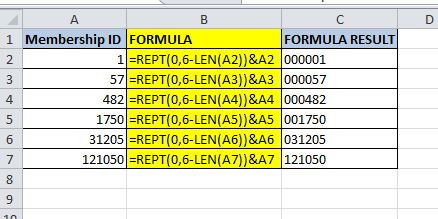Now the membership IDs are all 6 digits in length with the correct amount of leading zeros added, objective achieved

Summary

Adding leading zeros can be a common practice when creating datasets that contain unique IDs such as membership numbers or staff numbers.

There are two ways that you can add leading zeros, the first and easiest is to format the existing number. This will result in the value staying as a numeric.

The second way to format ensures you also convert the value to text which can have benefits depending on your task. To convert to text and add the leading zeros you need to utilise Excels REPT and LEN function.

Hopefully, you can now add leading zeros to a value with ease in your future Excel work.